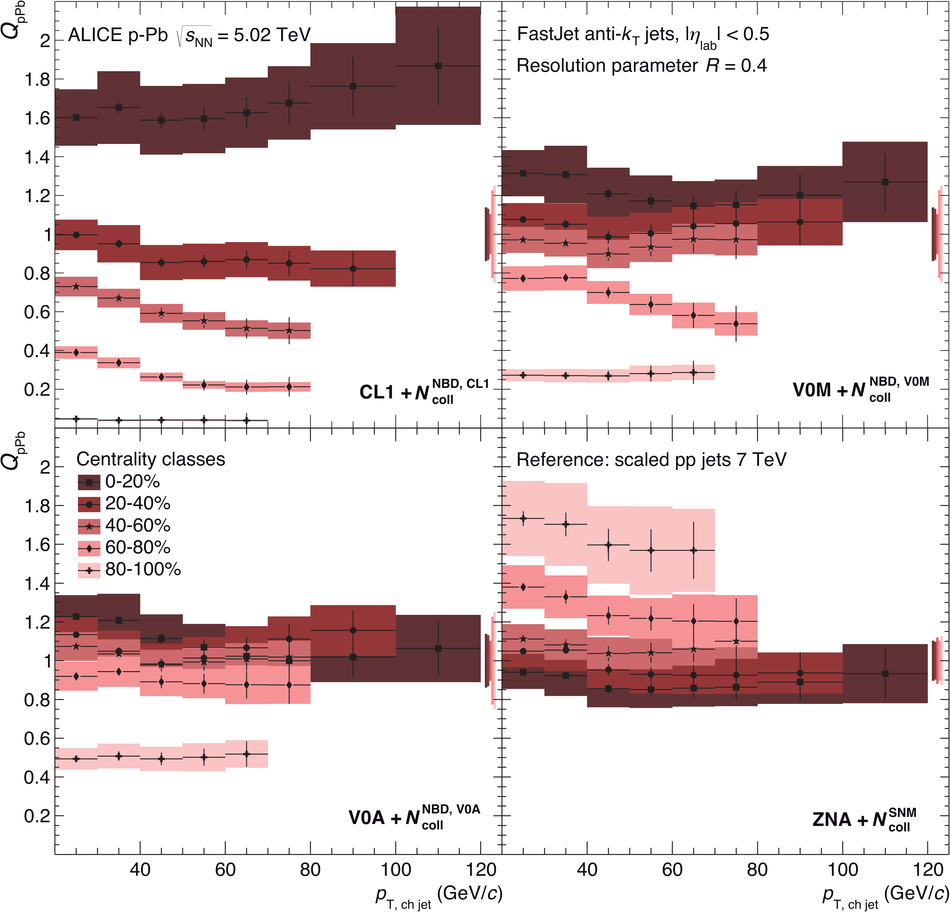# Centrality dependence of charged jet production in p-Pb collisions at $\sqrt{s_\mathrm{NN}}$ = 5.02 TeV

Measurements of charged jet production as a function of centrality are presented for p-Pb collisions recorded at $\sqrt{s_{\rm NN}} = 5.02$ TeV with the ALICE detector. Centrality classes are determined via the energy deposit in neutron calorimeters at zero degree, close to the beam direction, to minimise dynamical biases of the selection. The corresponding number of participants or binary nucleon-nucleon collisions is determined based on the particle production in the Pb-going rapidity region. Jets have been reconstructed in the central rapidity region from charged particles with the anti-$k_{\rm T}$ algorithm for resolution parameters $R = 0.2$ and $R = 0.4$ in the transverse momentum range 20 to 120 GeV/$c$. The reconstructed jet momentum and yields have been corrected for detector effects and underlying-event background. In the five centrality bins considered, the charged jet production in p-Pb collisions is consistent with the production expected from binary scaling from pp collisions. The ratio of jet yields reconstructed with the two different resolution parameters is also independent of the centrality selection, demonstrating the absence of major modifications of the radial jet structure in the reported centrality classes.

Figures

## Figure 1

 Left: Centrality dependence of the background momentum density $\rho_\mathrm{ch}$ determined with $\kt$ jets and $R = 0.4$. Right: $\delta_{p_{\rm T},{\rm ch}}$ distributions for different centralities obtained with random cones and $R = 0.4$.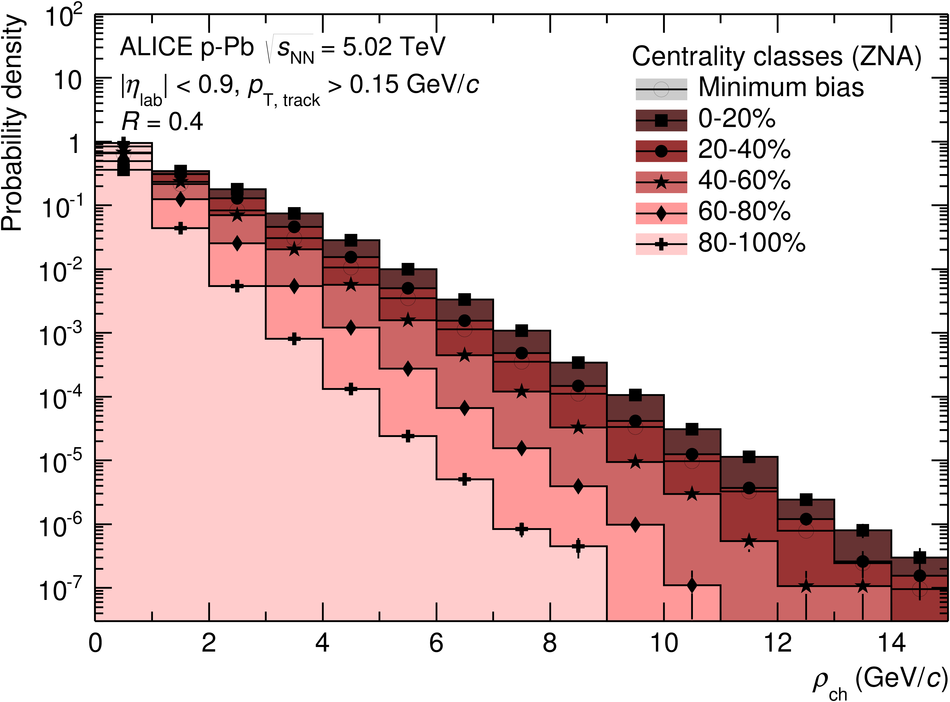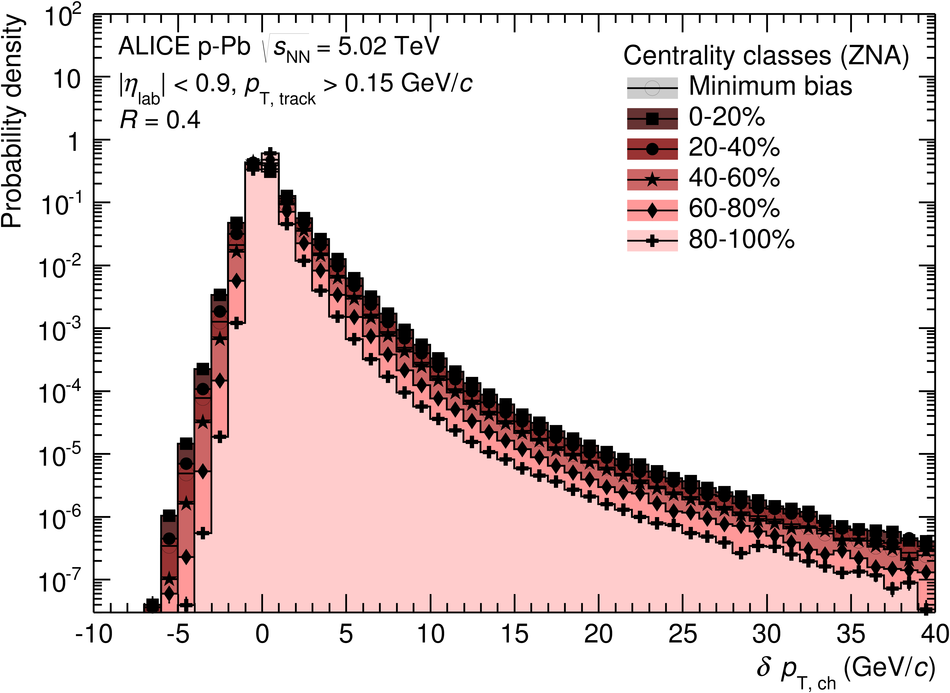## Figure 2

 $\pt$-differential production cross sections of charged jet production in p-Pb collisions at $5.02$ TeV for several centrality classes. Top and bottom panels show the result for $R=0.4$ and $R=0.2$, respectively. In these and the following plots, the coloured boxes represent systematic uncertainties, the error bars represent statistical uncertainties The overall normalisation uncertainty on the visible cross section is $3.3\%$ in p-Pb. The corresponding reference pp spectrum is shown for both radii, it was obtained by scaling down the measured charged jets at 7 TeV to the reference energy.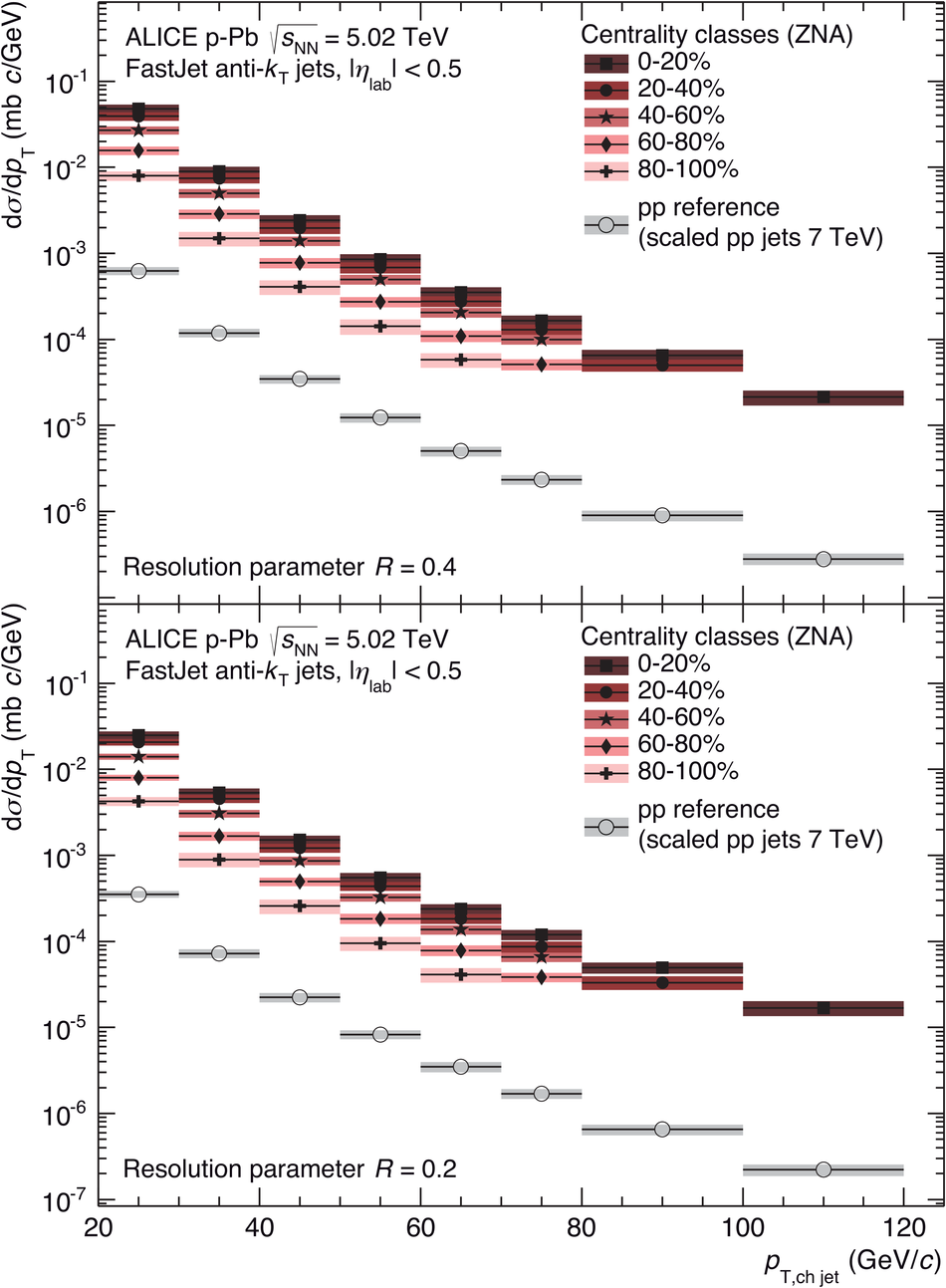## Figure 3

 Nuclear modification factors $Q_\mathrm{pPb}$ of charged jets for several centrality classes. $N_\mathrm{coll}$ has been determined with the hybrid model. Top and bottom panels show the result for $R=0.4$ and $R=0.2$, respectively. The combined global normalisation uncertainty from $N_\mathrm{coll}$, the measured pp cross section,and the reference scaling is indicated by the box around unity.## Figure 4

 Nuclear modification factor of charged jets compared to the nuclear modification factor for full jets as measured by the ATLAS collaboration . Note that the underlying parton $\pt$ for fixed reconstructed jet $\pt$ is higher in the case of charged jets.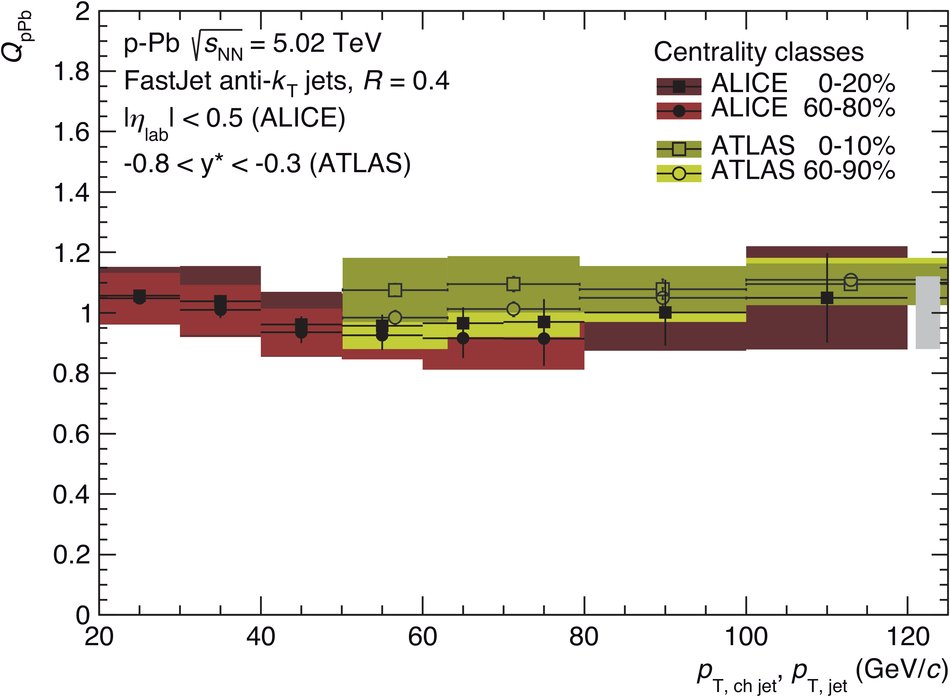## Figure 5

 Centrality evolution of $Q_\mathrm{pPb}$ for selected $\delta_{p_{\rm T},{\rm ch~jet}}$-bins and $R = 0.4$.## Figure 6

 Charged jet production cross section ratio for different resolution parameters as defined in Eq.8. Different centrality classes are shown together with the result for minimum bias collisions. Note that the systematic uncertainties are partially correlated between centrality classes. The ratio for minimum collisions is compared in more detail to pp collisions at higher energy and NLO calculations at $\sqrt{s} = 5.02$ TeV in , where no significant deviations are found.Centrality evolution of $Q_\mathrm{pPb}$ at fixed charged jet mometum for $R=0.4$. The V0-based centrality shows a clear dependence due to kinematical bias and the SNM estimate of $N_\mathrm{coll}$ is not realiable as discussed in arXiv:1412.6828.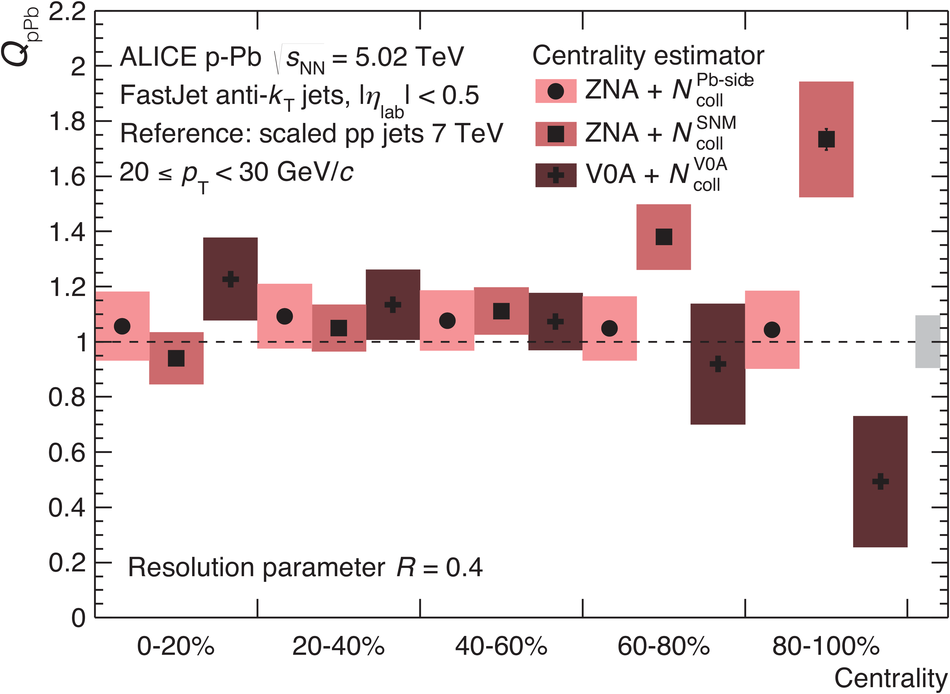$Q_\mathrm{pPb}$ of charged jet production in p-Pb for several different centrality classifications and $N_\mathrm{coll}$ estimates. Corresponding to Fig.19 for charged particles in arXiv:1412.6828.